## 一、背景介绍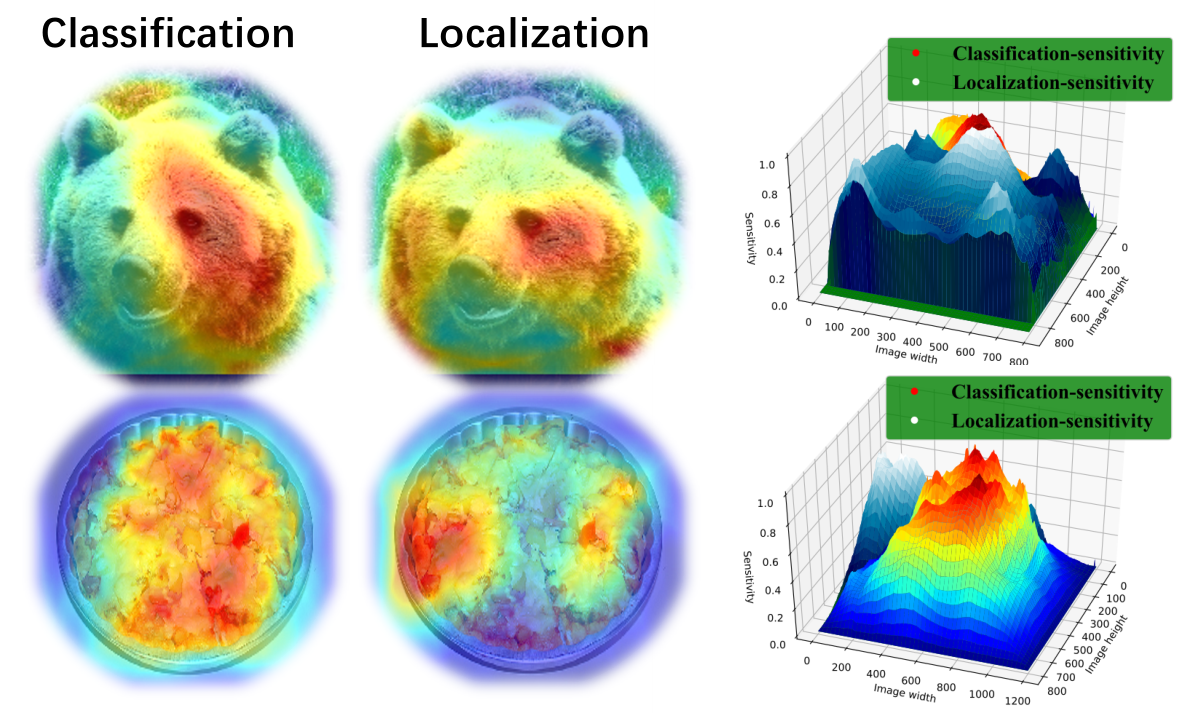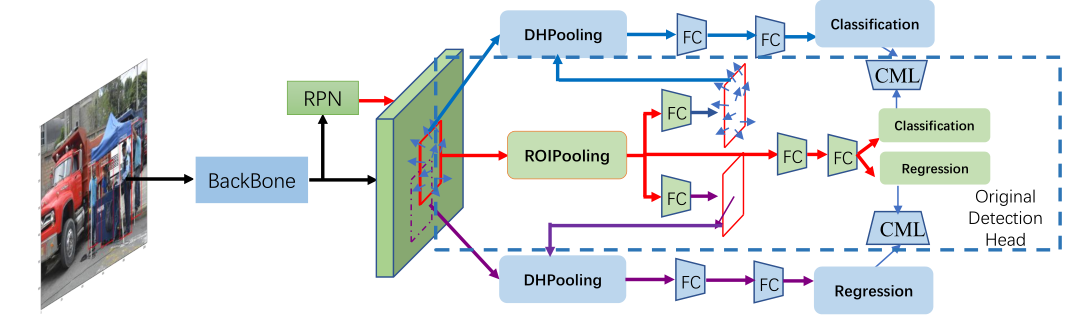## 二、 Task-aware spatial disentanglement learning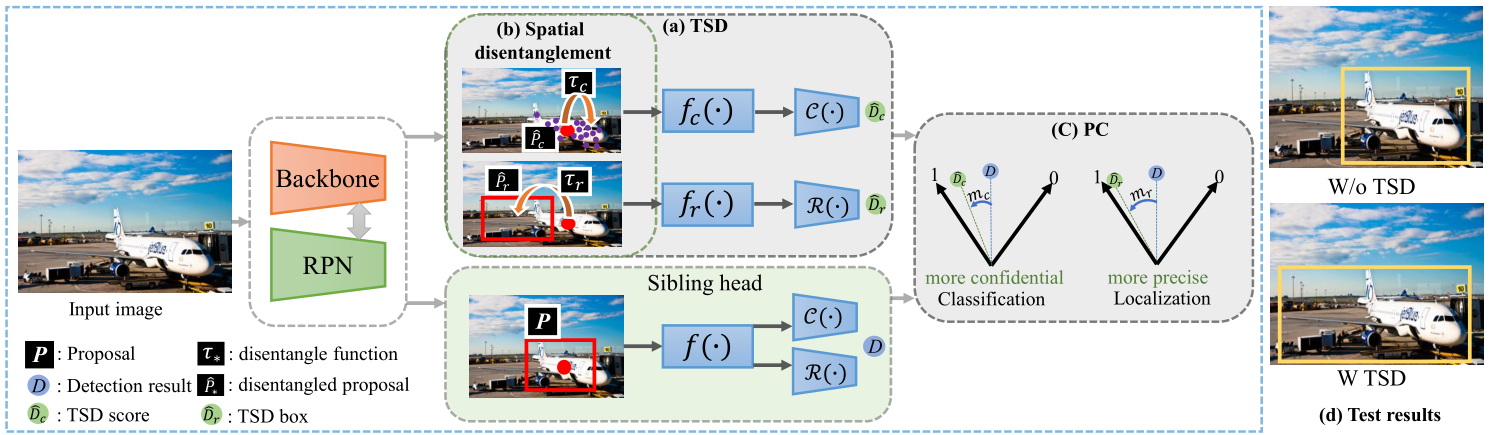$$\begin{array}{l} L = L_{cls}^D\left( {H_1^D\left( {{F_l},{{\hat P}_c}} \right),y} \right) + L_{loc}^D\left( {H_2^D\left( {{F_l},{{\hat P}_r}} \right),B} \right)\\ \left\{ \begin{array}{l} H_1^D\left( \cdot \right) = \left\{ {{f_c}\left( \cdot \right),C\left( \cdot \right)} \right\}\\ H_2^D\left( \cdot \right) = \left\{ {{f_r}\left( \cdot \right),R\left( \cdot \right)} \right\} \end{array} \right. \end{array}$$

$$\left\{ \begin{array}{l} {{\hat P}_c} = {\tau _c}\left( {P,\Delta C} \right),\Delta C = \gamma {F_c}\left( {F;{\theta _c}} \right) \cdot \left( {w,h} \right)\\ {{\hat P}_r} = {\tau _r}\left( {P,\Delta R} \right),\Delta R = \gamma {F_r}\left( {F;{\theta _r}} \right) \cdot \left( {w,h} \right) \end{array} \right.$$

## 三、 Progressive constraint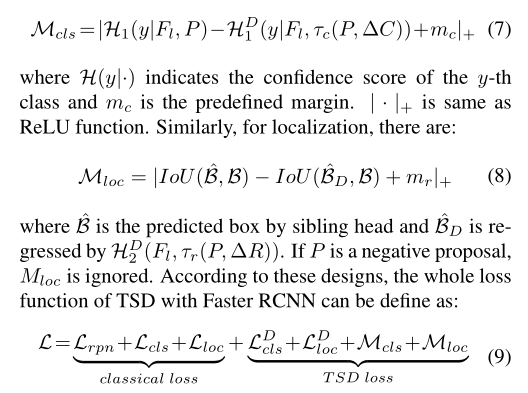## 四 、实验结果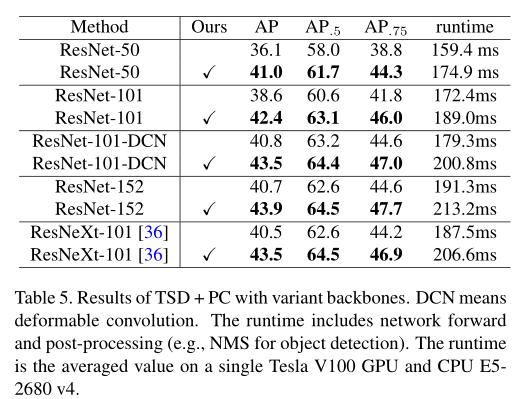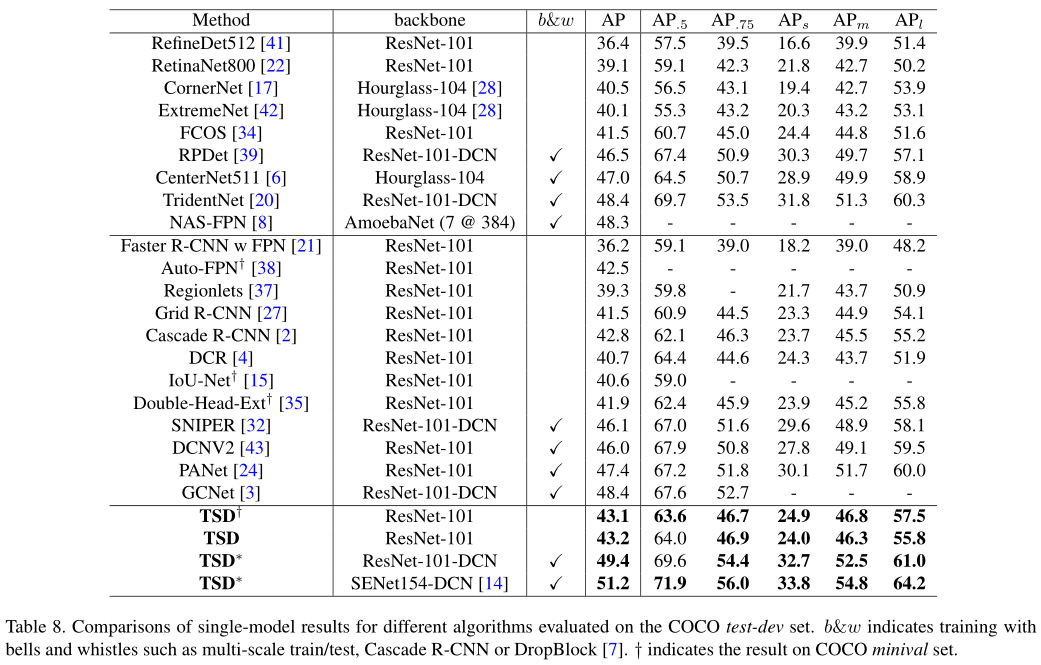## 五、总结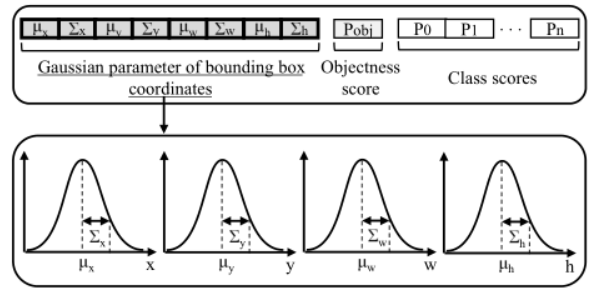$$prob = {P_{object}} \times {P_{clas{s_i}}} \times \left( {1 - \frac{{{\delta _x} + {\delta _y} + {\delta _w}{\rm{ + }}{\delta _h}}}{4}} \right)$$

## 参考资源

 Revisiting the Sibling Head in Object Detector

1st Place Solutions for OpenImage2019 - Object Detection and Instance Segmentation.

Gaussian YOLOv3: An Accurate and Fast Object Detector Using Localization Uncertainty for Autonomo

-------------本文结束感谢您的阅读-------------